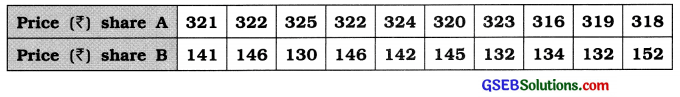# GSEB Solutions Class 11 Statistics Chapter 4 Measures of Dispersion Ex 4.5

Gujarat Board Statistics Class 11 GSEB Solutions Chapter 4 Measures of Dispersion Ex 4.5 Textbook Exercise Questions and Answers.

## Gujarat Board Textbook Solutions Class 11 Statistics Chapter 4 Measures of Dispersion Ex 4.5

Question 1.
Price fluctuations of two shares A and B are given below, which type of share has more relative variation in its price?To determine which share price has more relative variation, we calculate coefficient of variation of prices of share A and share B.Share A
Mean:
x̄ = $$\frac{\Sigma x}{n}=\frac{3210}{10}$$ = ₹ 321

Standard deviation:
s = $$\sqrt{\frac{\Sigma(x-\bar{x})^{2}}{n}}$$
= $$\sqrt{\frac{70}{10}}$$
= √7
= ₹ 2.65

Coefficient of variation:
Variation = $$\frac{s}{\bar{x}}$$ × 100
= $$\frac{2.65}{321}$$ × 100
= 0.0083 × 100
= 0.83%

Share B
Mean:
x̄ = $$\frac{\Sigma x}{n}=\frac{1400}{10}$$ = ₹ 140

Standard deviation:
s = $$\sqrt{\frac{\Sigma(x-\bar{x})^{2}}{n}}$$
= $$\sqrt{\frac{510}{10}}$$
= √51
= ₹ 7.14

Coefficient of variation:
Variation = $$\frac{s}{\bar{x}}$$ × 100
= $$\frac{7.14}{140}$$ × 100
= 0.051 × 100
= 5.1%

Coefficient of variation of price of share A 0.83% and that of share B it is 5.1%. Hence, the relative measure of variation is more in the price of share B.Question 2.
The daily salary of administrative staff of two companies yielded the following results:

 Company A Company B Mean salary(₹) 600 2100 Standard Deviation (₹) 30 84

Which company has more stable salary?
Company A
x̄ = ₹ 600
s = ₹ 30

Coefficient of variation = $$\frac{s}{\bar{x}}$$ × 100
= $$\frac{30}{600}$$ × 100
= 5%

Company B:
x̄ = ₹ 2100
s = ₹ 84

Coefficient of variation = $$\frac{s}{\bar{x}}$$ × 100
= $$\frac{84}{2100}$$ × 100
= 4%

The coefficient of variation of daily salary of employees of company A is 5 % and that of company B it is 4 %. Hence, the daily salary in company B Is more stable.

Question 3.
The Coefficients of variation of two series are 30% and 25% and their standard deviations are 15 and 9 respectIvely. Find their means.
First Series:
Coefficient of variation: 30%
s = 15
x̄ = ?

Coefficient of variation = $$\frac{s}{\bar{x}}$$ × 100
∴ 30 = $$\frac{15}{\bar{x}}$$ × 100
∴ 30x̄ = 1500
∴ x̄ = $$\frac{1500}{30}$$
∴x̄ = 50

Second series:
Coefficient of variation: 25%
s = 9
x̄ = ?

Coefficient of variation = $$\frac{s}{\bar{x}}$$ × 100
∴ 25 = $$\frac{9}{\bar{x}}$$ × 100
∴ 25x̄ = 900
∴ x̄ = $$\frac{900}{25}$$
∴x̄ = 36